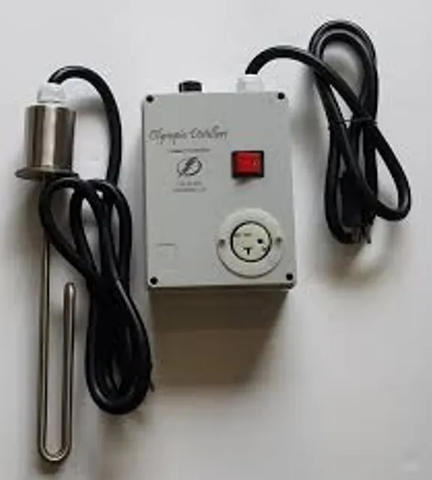• Oct 28, 2020
• by MoonshinerWelcome. We did a little bit of testing because I wanted to make a determination to at least give everybody a data point on heater elements that we use in stills or in brewing equipment. And the data point gives us an idea of where to start. So what I did was I took the three-gallon, I have, and tested three different elements. I tested the 1500 watt element, at 120 volts. I tested the 2000 watt element at 120 volts and I tested the 330 400 watt element, and I did that one at 240 volts. And what I tried to determine was what was the time lapse between the start to boil on all three of those.

These are those results that I was talking about. I have the 1500 watt element now I had that wired at 120 volts. I also had a 2000 watt element wired at 120 volts, but for the 3500-watt element I wired that one at 240 volts. So I wanted to see what the difference was between the three and a start. It was 78 degrees Fahrenheit on all three tests. And I measured it from that to a boil and the time in minutes it took to reach that boil.

You'll notice the blue line. That is a 1500 watt element. You can see how gradual that is. It took 37 minutes in order for me to achieve a boil. The 2000 watt elements spiked and then kind of leveled off at 30 minutes to a boil. You notice the green line. This is that 3500-watt element at 240 volts that only took 13 minutes which is what I kind of expected.

That gives you a data point on the efficiencies of 120 volts versus 240 volts and also the different sizes of elements. This was in a three-gallon kettle and remember you can always wire this 3500 water element at 120 volts but if you did you only get 25% of the wattage which is 875 watts. Now that you've seen that it can give you an idea and we use a three-gallon kettle, arm. Now when you wire these I want you to notice here see there's only two wires on the back of there that go you got one black and one white, or one black and one white. Your third wire is a ground wire and the ground wire goes to the side of the pot. And the reason the ground wires there that should something electrical happen in short take place and electrifies the pot at least has got somewhere to go without electrifying the pot, would you grab it? This one is actually wired exactly the same way, but it's hidden inside this coupling, and there are your three prongs.

So that's 120 volts. This is my 3400 watt. And this is a 240-volt element but a wired in 120 volts. I've got a black one here and a white one here, and I've got my ground right here. Remember what we said about that a 3500 watt element. It's 3500 watts 3500 times point two, five.

All I'm getting out of that is 875 watts of power. So it's one-fourth of the power if I run it on 120 volts, which I did, I just wired up to 120 volts. So that's what you got to keep in mind.

Now, remember, if I did this at 240 volts, the difference is that I have a black wire, which is the hot and a white wire which we call the neutral, open, here's my ground wire slipped off, If this was 240 volts, I would have a black wire that is 120 volts. And I would have a red wire in most cases, which is 120 volts. It doesn't matter which one of these screws you put it on, but you add those 220 volts together and you get to 40. And the reason you get 240s because they are 180 degrees out of phase.

You'll also have groundwater in the groundwater and you'll probably have a neutral wire now some 240-volt wires come four wires or three wires. If it's four wires, you'll have that extra wire. Where's that extra wire go? Well, you could put that right here with the ground because the ground and neutral go to the same place.  Until next time, we're gonna talk about pulse wave modulation efficient ways of checking.

We're gonna talk about pulse wave modulation efficient ways of checking next time!